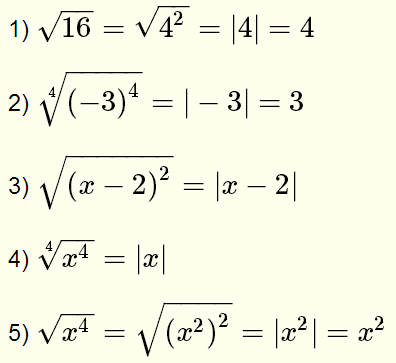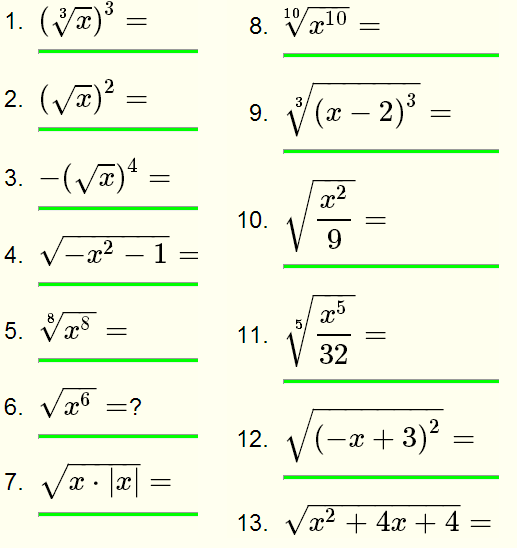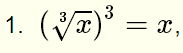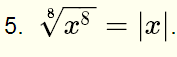Grade 10 questions on how to use some important formuals to simplify radicals algebraic expressions with solutions are presented.

Important Formulas
A) If n and m are positive integers and n√y is a real number, thenExamples
1) √5 is a real number and therefore2) 3√(-7) is a real number and thereforeB) If n is an EVEN positive integer thenExamplesC) If n is an ODD positive integer thenExamplesRewrite, if possible, the following expressions without radicals (simplify)Solutions to the Above ProblemsThe index of the radical 3 is odd and equal to the power of the radicand.since √x is a real number, x is positive and therefore |x| = x.is not a real number since -x2 - 1 is always negative..
The index 8 is even and equal to the power of the radicand....
The index 10 of the radical is even and equal to the power of the radicand..
The index 3 of the radical is odd and equal to the power of the radicand...Even index and power of radicand.Even index and power of radicand.

More High School Math (Grades 10, 11 and 12) - Free Questions and Problems With Answers
More Middle School Math (Grades 6, 7, 8, 9) - Free Questions and Problems With Answers
More Primary Math (Grades 4 and 5) with Free Questions and Problems With Answers
Author - e-mail